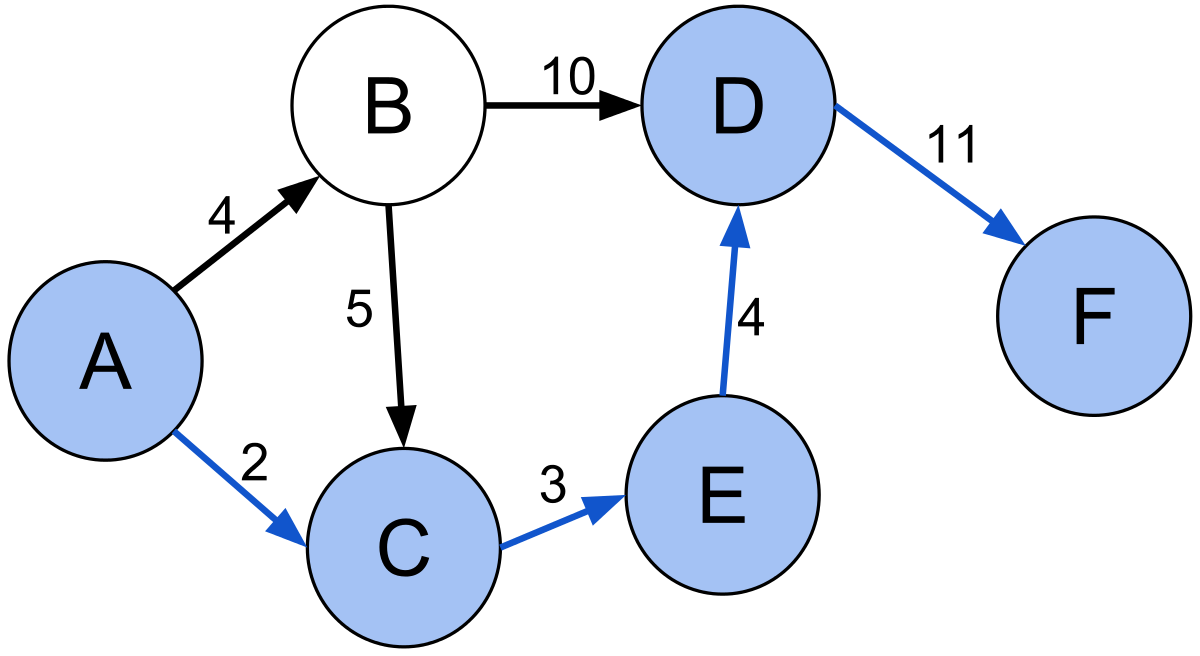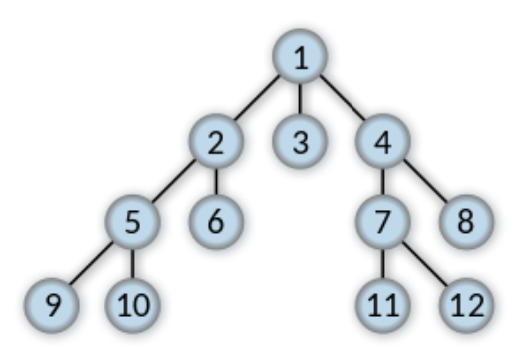# Learning Python with Advent of Code Walkthroughs

Dazbo's Advent of Code solutions, written in Python

# The Python Journey - Shortest Path Algorithms - BFS, Dijkstra, and A*## Overview

Honestly, you won’t get far in Advent-of-Code if you don’t know them. Eric loves challenges like…

• Find the shortest path from a to b
• Find the optimum way to arrange this thing

Here are my three top reasons why you should learn this:

1. You’ll get the right answer!
2. It’ll save you LOADS of time and effort.
3. These algorithms are cool!!

## Algorithm Summary

First, let me provide a quick summary and comparison. Then we’ll get into the details after.

Algorithm Summary When To Use It
Breadth First Search (BFS) Aka "flood fill". Explores equally in all directions. Uses a FIFO queue. A `list` will work, but a `deque` is best. For finding the shortest path where the _cost_ is constant for any move. Or, for finding every point in a graph. Or for finding ALL paths from a to b.
Dijkstra’s Algorithm Flood fill + a cost-based optimisation. I.e. prioritises paths to explore, favouring lower cost paths. Uses a _priority queue_, i.e. `heapq`. When movement costs may vary.
A* Optimised for a single destination. I.e. prioritises paths that _seem_ to be approaching the goal. Still uses a `heapq`, but uses some sort of cost heuristic. Depending on the value of the distance heuristic, it may not add much value over Dijkstra, and may actually be slower. When we have an idea of the direction we need to follow.

## The Final Frontier!

All these algorithms utilise the concept of an expanding frontier. This expanding frontier is sometimes called a flood fill. It gives us a way to visit every allowed location on a map.

How the frontier works:

1. Pick and remove a location from the frontier. Let’s call it current.
2. Expand the frontier by finding all of the valid next moves from current. Let’s call them neighbours. Add the neighbours to the frontier.

Here’s some code that will perform a flood fill to explore every valid location in the graph:

``````frontier = Queue() # For BFS, we just use a FIFO queue.
frontier.put(start)
explored = set()  # To stop us exploring the same location more than once.

# keep going until the frontier is empty; i.e. when we've explored all the valid nodes
while frontier:
current = frontier.popleft()  # pop the first item off the FIFO queue
for next in graph.neighbors(current):  # get all the valid neighbours
if next not in explored:
frontier.put(next) # add it to the frontier
explored.add(next)  # mark it as explored
``````

BFS can be used to find a path from a to b. It can also be used to find paths from a to all locations in the graph.

It is guaranteed to find a solution if one exists. The frontier expands at an equal rate in all directions, and uses a FIFO (first-in, first-out) queue to implement the frontier.

### How It Works

• We want to explore a graph, made up of edges connected by vertices. E.g. if we’re solving the path through a map, then each map location is a vertex. The graph has a start location, which is the root node of our tree. So we add the root to the frontier.
• It then explores all adjacent nodes. These will be `distance=1` from the root. We add those to the frontier.
• Then we explore all the nodes that are adjacent to those nodes. I.e. the nodes that are `distance=2` from the root.
• The BFS algorithm ensures that the same vertex is not followed twice; i.e. we never revisit a node we’ve visited before. This is crucial because it means that once we’ve a path to a note, we must have found the shortest route to that node.

So the sequence of exploration looks like this:The numbers represent the sequence of traversal. But note that it is possible for the same node to appear in the tree more than once.

When we find a node that matches our goal, we stop. Thus, a BFS is just a flood fill, with a goal.

### The Queue

We can implement the queue in many ways:

• Using a vanilla `list`.
• Better: using a `deque` to implement a FIFO queue; it is much more efficient for appending and popping at each end.
• If we use a `heapq`, then we end up popping based on a priority rather than FIFO. This is how we implement Dijkstra’s Algorithm or A*.
• If we use a `stack`, then we end up with last-in, first-out (LIFO), which actually turns a BFS into a depth first search (DFS)!

### Implementing the BFS

Here’s some pseudocode that shows how to implement the BFS:

``````function BFS(graph, root):
queue = new Queue()
queue.enqueue(root)
while queue not empty:
current = queue.pop()
if current is goal:
return current

if neighbour is not explored:
label neighbour as explored
queue.enqueue(neighbour)
``````

There are a couple of convenient ways to label a node as explored:

1. We can store them in a `set`.
2. We can add them to a `dictionary`

If we use a `set`:

``````frontier = deque() # For BFS, we need a FIFO queue. A deque is perfect.
frontier.append(start)
explored = set()  # To stop us exploring the same location more than once.
explored.append(start)

# keep going until the frontier is empty; i.e. when we've explored all the valid nodes
while frontier:
current = frontier.popleft()  # pop the first item off the FIFO queue

if current == goal:
break

for next in graph.neighbors(current):  # get all the valid neighbours
if next not in explored:
frontier.put(next) # add it to the frontier
explored.add(next)  # mark it as explored

if current != goal:
# there was no solution!
``````

### Keeping Track of Distance

If we’re only interested in the shortest distance to a point, but we don’t need to create the actual path, a cool trick is to keep track of the distance in our queue. E.g.

``````frontier = deque() # For BFS, we need a FIFO queue. A deque is perfect.
frontier.append(start, 0) # Queue always includes (point, steps)
explored = set()  # To stop us exploring the same location more than once.
explored.append(start)

# keep going until the frontier is empty; i.e. when we've explored all the valid nodes
while frontier:
current, steps = frontier.popleft()  # pop the first item off the FIFO queue

if current == goal:
break

for next in graph.neighbors(current):  # get all the valid neighbours
if next not in explored:
frontier.put(next, steps+1) # add it to the frontier
explored.add(next)  # mark it as explored

if current != goal:
# there was no solution!

# distance from start to goal = steps
``````

If we use a `dictionary` instead of a `set`, we can create a breadcrumb trail to each point we’ve explored.

Let’s explore every valid point in the graph:

``````frontier = deque() # For BFS, we just use a FIFO queue. A deque is perfect.
frontier.append(start)
came_from = {}  #  To stop us exploring the same location more than once; and for breadcrumbs
came_from[start] = None  # This marks the last breadcrumb

# keep going until the frontier is empty; i.e. when we've explored all the valid nodes
while frontier:
current = frontier.popleft()  # pop the first item off the FIFO queue

for next in graph.neighbors(current):  # get all the valid neighbours
if next not in came_from:
frontier.put(next) # add it to the frontier
came_from[next] = current
``````

We can then create the breadcrumb path, like this:

``````current = goal  # start at the end
path = []       # to store every point in a specific path
while current != start:
path.append(current)
current = came_from[current]  # get the next node in the trail
path.append(start) # optional - depends if we want the first node to be included or not
path.reverse() # optional - depends whether we want start->end or end->start
``````

## A* Algorithm

The A* Algorithm is a lot like BFS. But instead of expanding frontier in all directions equally - called an unweighted pathfinder algorithm - we prioritise popping from the frontier in the direction that we believe takes us closer to our goal. Thus, a weighted pathfinder algorithm.

To do this, we need two key changes, compared to the BFS:

1. We need to use a `heapq`. Recall that a `heapq` allows us to pop elements based on a priority.
2. We need to provide a heuristic function, i.e. a function that computes some sort of cost. The heuristic estimates the cost to get from any given node to the goal. This cost is used to determine the priority. Typically, the heuristic will be a function that estimates distance, such as a Manhattan distance function.

### Turning a BFS into an A*

Here’s a BFS that creates a breadcrumb path. The priority of the pop is simply the number of steps taken from the starting point.

``````def solve_with_bfs(start:Point, goal:Point) -> tuple[int, dict]:
queue = []
heapq.heappush(queue, (0, start)) # posn, steps
came_from = {} # use a dict so we can build a breadcrumb trail
came_from[start] = None

while queue:
step_count, curr_posn = heapq.heappop(queue)

# Check if the target is reached
if curr_posn == goal:
return step_count, came_from

for dx, dy in Hex.hex_vectors.values():
adjacent = curr_posn + Point(dx, dy)

assert False, "We should never get here."
``````

Here I’ve changed the code to an A*. Now I’m popping from the `heapq`, using a priority that is sum of the number of steps taken, and the estimated distance to the goal.

``````def solve_with_astar(start: Point, goal: Point) -> tuple[int, dict]:
""" Obtain the fastest path from origin to goal using A*.
Returns: tuple[int, dict]: steps required, breadcrumbs
"""
queue = []
came_from = {} # use a dict so we can build a breadcrumb trail
came_from[start] = None
heapq.heappush(queue, (0, 0, start)) # distance heuristic, steps, posn

while queue:
_, step_count, curr_posn = heapq.heappop(queue)

# Check if the target is reached
if curr_posn == goal:
return step_count, came_from

for dx, dy in Hex.hex_vectors.values():
adjacent = curr_posn + Point(dx, dy)